Mathematics and Computer Science
Volume 1, Issue 3, September 2016, Pages: 48-55

Discussion on Borehole Wave Modes Excited by Two Types of Acoustic Logging While Drilling Sources

Kuo Zhang1, 2

1Beijing CCID Publishing & Media Co., Ltd., Beijing, China

2State Key Laboratory of Petroleum Resources and Prospecting, China University of Petroleum, Beijing, China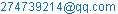Kuo Zhang. Discussion on Borehole Wave Modes Excited by Two Types of Acoustic Logging While Drilling Sources. Mathematics and Computer Science. Vol. 1, No. 3, 2016, pp. 48-55. doi: 10.11648/j.mcs.20160103.13

Received: September 6, 2016; Accepted: September 19, 2016; Published: September 29, 2016

Abstract: In this paper, we extend the Real Axis Integration (RAI) method and improve the Finite Difference Time Domain (FDTD) method to investigate the wave fields excited by the monopole and multipole Acoustic Logging While Drilling (ALWD) sources with low and high center frequencies. We simulate the two traditional kinds of source exerting methods by both of the RAI and FDTD methods accurately and efficiently. Mutual verification of the two methods ensures the validity and reliability of our theoretic analysis and modeling results. The modeling results indicate that the ring source can only excite those monopole or multipole wave modes of certain orders. The four azimuthally orthogonal point sources can excite similar wave modes only at the lower frequencies, but at higher frequencies, they might further excite wave modes of higher orders with significant amplitude. These modeling results may help the design of ALWD tools, and also provide an essential basis for the further analysis of the ALWD problems in anisotropic formations and tool eccentric conditions.

Keywords: Logging While Drilling, Real Axis Integration, Finite Difference Time Domain, Staggered Grid Scheme, Perfectly Matched Layer, Ring Source, Azimuthally Orthogonal Point Sources, Multipole Wave Mode

Contents

1. Introduction

Logging While Drilling (LWD) technologies, including Acoustic Logging While Drilling (ALWD), have attracted increasingly attentions in recent years [1-2]. However, the ALWD tool is affected by more complex environments than the wireline tool [3-5]. Therefore, much of the theoretical analysis for the wireline case cannot be directly applied to the ALWD case. It is necessary to develop new numerical models to simulate the ALWD responses under field conditions. The most common forward modeling methods include the semi-analytical methods such as Real Axis Integration (RAI), and numerical methods such as Finite Difference Time Domain (FDTD) and Finite Element Method (FEM). Of these three methods, RAI needs the least computer resources, but it is only applicable to the simple cases of symmetrical and regular geometry. Because there are previously published results that have been well recognized in this research field, it can be readily used as a standard reference for numerical modeling in these simple cases. The FDTD method is relatively rapid and can simulate some complicated models. In addition, the widely employed Staggered Grid Scheme (SGS) of the FDTD method can be adapted for media having different Poisson’s Ratios . It has thus been widely adopted in borehole acoustic modeling. However, the accuracy of such an SGS of the FDTD method cannot always be guaranteed due to the complex structure of ALWD tools . Although the FEM is of higher accuracy and can be well adapted for complicated models, the huge inherent computational cost makes it very difficult to solve high frequency 3D problems, even with a super computer . Under such considerations, in this paper, we first extend the applicability of the RAI method and modify the SGS to improve the accuracy of the FDTD method for ALWD modeling, and then apply the improved methods for the analysis of the borehole wave modes excited by two types of ALWD sources.

2. Basic Theory and Modeling Methods

2.1. Physical Model

Figure 1 shows the two traditional kinds of sources in ALWD modeling . The positions of both sources are on the boundary between the collar and the outer fluid. The parameters of our forward modeling in such cases are shown in Table 1.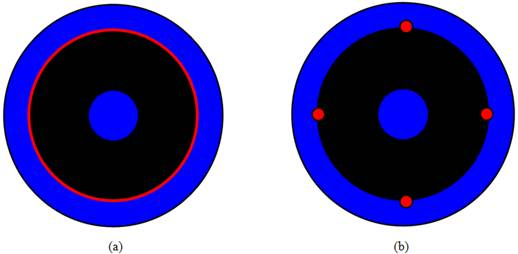Figure 1. A general view of the two traditional kinds of sources in ALWD modeling. ((a) the ring source; (b) the four azimuthally orthogonal point sources.) The blue color represents the borehole fluid, the black the collar, and the red the source exerting methods.

Table 1. The parameters of forward modeling.

 P-wave velocity Vp / (m/s) S-wave velocity Vs / (m/s) Density ρ / (kg/m3) Radius r /m Inner fluid 1470 0 1000 0.027 Collar 5860 3300 7850 0.090 Outer fluid 1470 0 1000 0.117 Fast formation 3972 2455 2320 Inf Slow formation 2300 1000 2000 Inf

2.2. Mathematical Model of RAI

The mathematical expression of the RAI method is given below,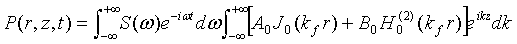(1)

where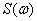is the spectrum of the source function;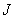and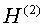are the Bessel function of the first kind and the Hankel function of the second kind, respectively. The unknown coefficients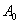and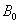depend on the boundary conditions of the model.

For corresponding dispersion curves, there are poles at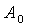and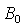, therefore the numerical integral about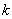will not be accurate and a complex frequency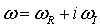is introduced to bypass these poles. The complex frequency introduced can cause an artificial attenuation of the waves in the time domain, so the waves must be dot-multiplied by a coefficient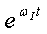to compensate for the attenuation. However, the effects due to the nonlinear characteristics of the RAI equation become more unpredictable as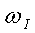becomes very large, and can no longer be compensated by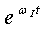. A trial-and-error method should be exercised carefully to ensure correct results .

We can directly obtain the results of the ring source case with Equation (1). However, it is more complex if we need to solve the case of four azimuthally orthogonal point sources. Without loss of generality, consider one of the eccentric point sources by the Bessel Additional Theorem,(2)

where,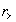and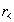are the bigger and the smaller between the radial distance of the source and the receiver. The borehole wave field is a summation of the contributions by the four azimuthally orthogonal point sources .

2.3. Numerical Model of FDTD

Figure 2 shows the xy surface of our FDTD ALWD modeling. The detailed parameters are as follows: the size of the model is 0.3 m by 0.3 m by 3.6 m. The offset is 2 m, and the spacing between the 8 receivers is 0.15 m. The grid size is 0.003 m, which suppresses the numerical dispersion preferable, guaranteeing that there are 9 grids in the thickest outer fluid so that it is well converged, and the sawteeth on the boundary is not so rough. There is a 10-grid Perfectly Matched Layer (PML) around the model to simulate the infinity domain. Based on the numerical stability condition, we choose the time step as 0.25 μs. By exerting the point sources to all of the nodes on the boundary of the collar and the outer fluid, it becomes a ring source. It is simple to model the four azimuthally orthogonal point sources and we no longer specially state them here.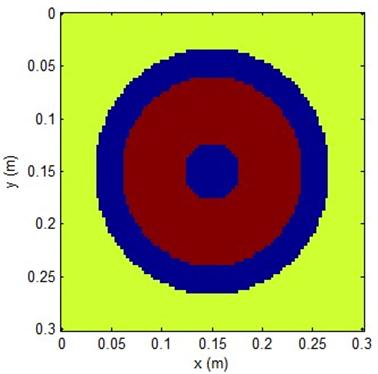Figure 2. The xy surface of the FDTD ALWD modeling. The blue color represents the borehole fluid, the brown the collar, and the yellow the formation.

Choosing a reasonable order of the finite difference is also an essential scheme in the FDTD modeling. The most commonly used orders are o (t2+x4), o (t2+x8), and so on. However, we found that the spatial difference of 4th order or more does not fit the ALWD model, because the characteristics of the media vary greatly. Consider one of the finite differences,(3)

where 9/8 and -1/24 are the 4th order difference coefficients. However, we should realize the premise of these coefficients is that the medium is homogeneous, but the variance between the modulus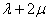of the collar and the borehole fluid is more than 100 times. As is shown in Figure 3, the finite differences around the boundary are no longer accurate, and when the difference order increases, there are more nodes affected by the error. In contrast to the traditional idea, and through some of our numerical attempts, we recognize that the spatial difference of the 2nd order can obtain a more satisfactory result.

The computation cost in each of our 3D FDTD ALWD modeling is about 3 GB in the computer memory and 4~5 hours in computation time. It is estimated that in the 3D FEM modeling, 300 GB or more of computer memory and 2 weeks or more of computation time will be necessary. So it would be advantageous if we can get the results that are perfect even by using the FDTD.Figure 3. An example to illustrate the inaccuracy of the finite differences around the boundary of fluid and collar. The x component finite difference of the black node needs the field values of the horizontal red nodes. In the domain with yellow color, the x component finite difference is inaccurate due to the severe inhomogeneity.

3. Validation and Discussion of Results

3.1. Monopole Waveforms

Figure 4 shows the waveforms excited by the monopole source with 10 kHz center frequency in the fast formation. Figure 4a shows the case of ring source and Figure 4b the case of four azimuthally orthogonal point sources. The arrival time, the amplitude and the phase of the two results are well coincident with each other, which indicates that the parameter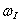in the RAI is well optimized and the sawteeth in the rectangular coordinate FDTD model can be negligible. The subsequent results also satisfy this conclusion and will no longer be stated again.

Figure 4a clearly shows that there are two wave trains, in which the sliding S-wave propagates as the velocity of the formation S-wave with lower amplitude, and the Stoneley wave propagates at near the velocity of the borehole fluid P-wave with larger amplitude. Besides, there are also collar and sliding P-waves that arrive earlier with even lower amplitudes, so they cannot be observed at this scale.

Being different from the case of ring source, Figure 4b shows that there are two more irregular wave trains after the arrival of the Stoneley wave. From an investigation of the RAI, the wave train of large amplitude after the Stoneley wave is the 4th order multipole wave mode (namely octapole) excited by the four eccentric azimuthally orthogonal point sources. After the octapole mode, and throughout the time scale, there are some weak vibrations, which are due to the 8th order multipole wave mode (namely hexadecapole).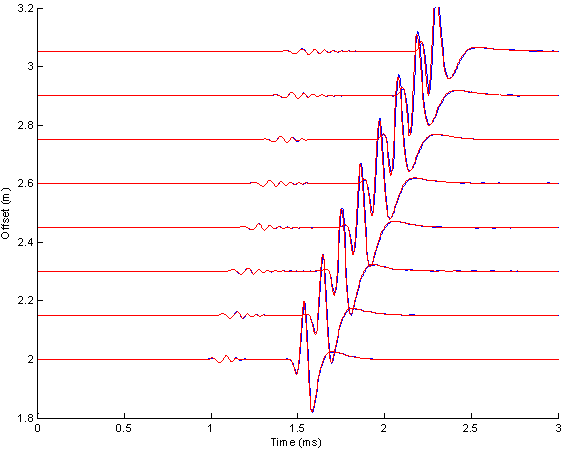(a)(b)

Figure 4. The waveforms excited by the monopole source with 10 kHz center frequency in the fast formation. (a) The case of ring source; (b) the case of four azimuthally orthogonal point sources. The red curve represents the RAI result, and the blue the FDTD result.

Figure 5 shows the waveforms excited by the monopole source with 10 kHz center frequency in the slow formation. Figure 5a shows the case of ring source and Figure 5b the case of four azimuthally orthogonal point sources.

Figure 5a clearly shows that there are two wave trains, in which the leaky P-wave propagates as the velocity of the formation P-wave with lower amplitude, and the Stoneley wave propagates at near the velocity of the borehole fluid P-wave with larger amplitude. Besides, there is also a collar wave that arrives earlier and its amplitude is even lower, thus cannot be seen at this scale. By Snell’s law, the sliding S-wave does not exist.

Being different from the case of ring source, Figure 5b shows that there is one more wave train after the arrival of the Stoneley wave. From the investigation of the RAI, it is the 4th order multipole wave mode (namely octapole) excited by the four eccentric azimuthally orthogonal point sources. The 8th order multipole wave mode (namely hexadecapole) and the higher orders are very weak in amplitude and can be negligible.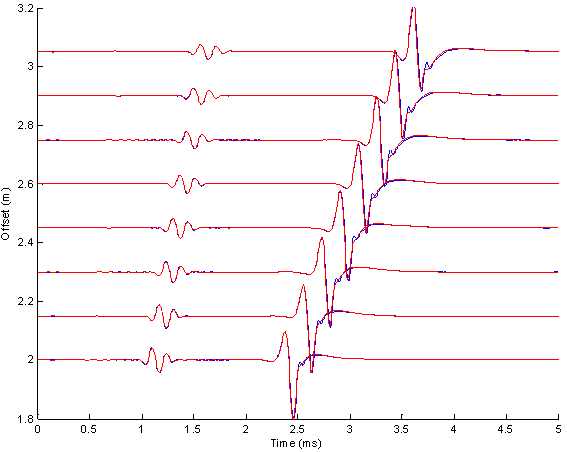(a)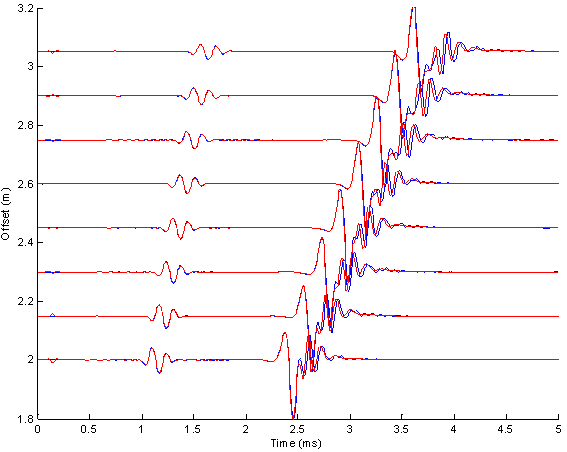(b)

Figure 5. The waveforms excited by the monopole source with 10 kHz center frequency in the slow formation. (a) The case of ring source; (b) the case of four azimuthally orthogonal point sources. The red curve represents the RAI result, and the blue the FDTD result.

3.2. Dipole Waveforms

Figure 6 shows the waveforms excited by the dipole source in the slow formation. Figure 6a shows the case of 2 kHz center frequency and Figure 6b the 10 kHz center frequency. In order to show the differences clearly between the two traditional source exerting methods, the red curve represents the RAI result of pure dipole mode, and the blue the FDTD result for the case of four azimuthally orthogonal point sources.

Figure 6a clearly shows that there are two wave trains, in which the collar flexural wave propagates at less than the velocity of the collar P-wave with lower amplitude, and the formation flexural wave propagates at less than the velocity of the formation S-wave with larger amplitude, thus being different from the wireline case, the dipole source in the ALWD model cannot excite the waveforms that are suitable for measuring the formation S-wave, which is consistent with some previous work [4,7,10]. Besides, because the center frequency of the source is low, the higher orders are not excited, thus the waveforms of the two source exerting methods are consistent with each other.

Being different from the case of 2 kHz center frequency, Figure 6b shows that, firstly, as the center frequency increases, the arrival times of the collar flexural wave and the formation flexural wave are much earlier, which is a further indication of the severe dispersion of the two wave modes; secondly, there are some obvious differences between the two results after the formation flexural wave arrival. From an investigation of the RAI, it is mainly the 3rd order multipole wave mode (namely hexapole) excited by this "special" dipole source. The 5th order multipole wave mode (namely decapole) and the higher orders are very weak in amplitude and can be negligible.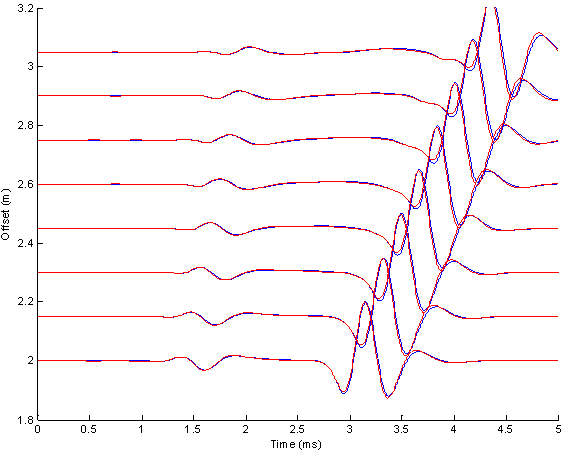(a)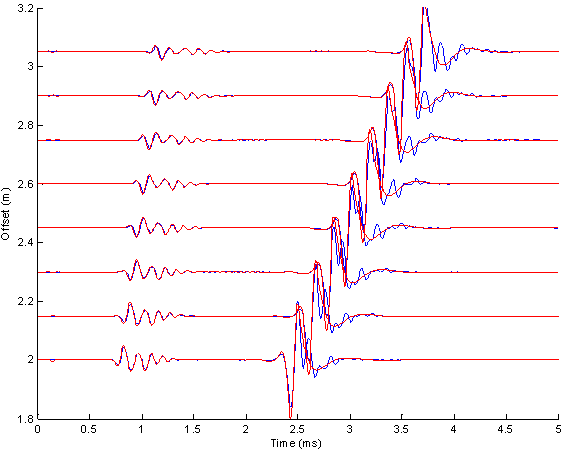(b)

Figure 6. The waveforms excited by the dipole source with (a) 2 kHz center frequency; (b) 10 kHz center frequency, in the slow formation. (The red curve represents the RAI result of pure dipole mode, and the blue the FDTD result for the case of four azimuthally orthogonal point sources.

Figure 7 shows the waveforms excited by the quadrupole source in the slow formation. Figure 7a shows the case of 2 kHz center frequency and Figure 7b the case of 10 kHz center frequency. As with the dipole case, we use the red curve to represent the RAI result of pure quadrupole mode, and the blue the FDTD result for the case of the four azimuthally orthogonal point sources.

Figure 7a clearly shows that there is only one wave train, which is the quadrupole wave that propagates at near the velocity of the formation S-wave, thus being similar to the wireline case, the quadrupole source in the ALWD model can excite the waveforms that are suitable for measuring the formation S-wave, which is also consistent with some previous work [4,7,10]. Besides, because the center frequency of the source is low, the higher orders are not excited, thus the waveforms of the two source exerting methods are consistent with each other.

Being different from the case of 2 kHz center frequency, Figure 7b shows that, firstly, as the center frequency increases, the collar wave appears with low and irregular amplitude, which indicates that the quadrupole source with high center frequency can no longer excite the waveforms that are suitable for measuring the formation S-wave; secondly, there are some slight differences between the two results after the quadrupole wave arrival. From an investigation of the RAI, it is mainly the 6th order multipole wave mode (namely dodecapole) excited by this "special" quadrupole source. The 10th order multipole wave mode (namely vigintipole) and the higher orders are very weak in amplitude and can be negligible.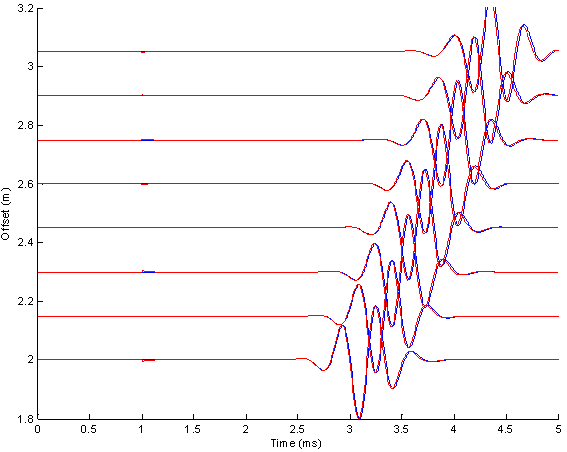(a)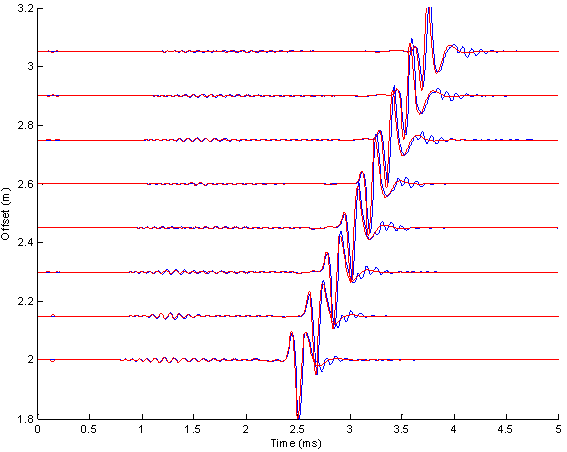(b)

Figure 7. The waveforms excited by the quadrupole source with (a) 2 kHz center frequency; (b) 10 kHz center frequency, in the slow formation. The red curve represents the RAI result of pure quadrupole mode, and the blue the FDTD result for the case of four azimuthally orthogonal point sources.

4. Conclusions

In this paper, we have extended the RAI method and improved the FDTD method to study the wave fields excited by monopole and multipole ALWD sources with low and high center frequencies. We have simulated the two types of source exerting methods by both of the RAI and FDTD methods accurately and efficiently. From these comprehensive simulations, we draw three conclusions below:

(1) The ring source can excite specific multipole wave mode of certain order;

(2) The four azimuthally orthogonal point sources can excite specific multipole wave mode of certain order only at the lower frequencies. At higher frequencies, however, they might further excite wave modes of higher orders with amplitudes which are not negligible;

(3) The modeling results in this paper may help a lot in the design of ALWD tools, and also provide an essential basis for a further analysis of the ALWD responses in anisotropic formations and tool eccentric conditions.

Acknowledgements

The author would like to thank Prof. Guo Tao from the Petroleum Institute in the United Arab Emirates for his linguistic assistance during the preparation of this manuscript, and Dr. Hua Wang from Massachusetts Institute of Technology in the United States for providing a forward simulator. The FDTD modeling results of this paper are based on a substantial improvement on this simulator by the author.

References

1. Wang T, Signorelli J. Finite-difference modeling of electromagnetic tool response for logging while drilling. Geophysics, 2004, 69 (1): 152-160.
2. Tang X M, Dubinsky V, Wang T, et al. Shear-Velocity Measurement in the Logging-While-Drilling Environment: Modeling and Field Evaluations. Petrophysics, 2003, 44 (2): 79-90.
3. Huang X J, Zheng Y B, Toksöz M N. Effects of tool eccentricity on acoustic logging while drilling (LWD) measurements. SEG Technical Program Expanded Abstracts, 2004: 290-293.
4. Wang B, Tao G, Chen T R. Experimental study of acoustic logging while drilling measurements using an asymmetric source. Chinese Journal of Geophysics, 2012, 55 (1): 327-332.
5.  Wang H, Tao G, Zhang K. Wavefield simulation and analysis with the finite-element method for acoustic logging while drilling in horizontal and deviated wells. Geophysics, 2013, 78 (6): D525-D543.
6. Wang T L, Tang X M. Finite-difference modeling of elastic wave propagation: A non-splitting perfectly matched layer approach. Geophysics, 2003, 68 (5): 1749-1755.
7. Wang H, Tao G, Wang B, et al. Wave field simulation and data acquisition scheme analysis for LWD acoustic tool. Chinese Journal of Geophysics, 2009, 52 (9): 2402-2409.
8. Yan X H. Numerical simulation investigation on acoustic transmission characteristics in Logging While Drilling. PhD Dissertation, China University of Petroleum, 2010.
9. Byun J M. Toksöz M N. Analysis of the acoustic wavefields excited by the Logging-While-Drilling (LWD) tool. Geosystem Engineering, 2003, 6 (1): 19-25.
10. Tang X M, Cheng A. Quantitative borehole acoustic methods. Elsevier, Volume 24 of Handbook of Geophysical Exploration, Seismic Exploration, 2004.
11. Zhang K, Tao G, Li J X, et al. On RAI and FDFE Simulations of Acoustic Well Logging Scheme. 75th EAGE Conference & Exhibition incorporating SPE EUROPEC, 2013.
12. Byun J, Toksöz M N. Effects of an off-centered tool on dipole and quadrupole logging. Geophysics,2006,71 (4): F91-F100.

 Contents 1. 2. 2.1. 2.2. 2.3. 3. 3.1. 3.2. 3.3. 4.
Article ToolsAbstractPDF(636K)